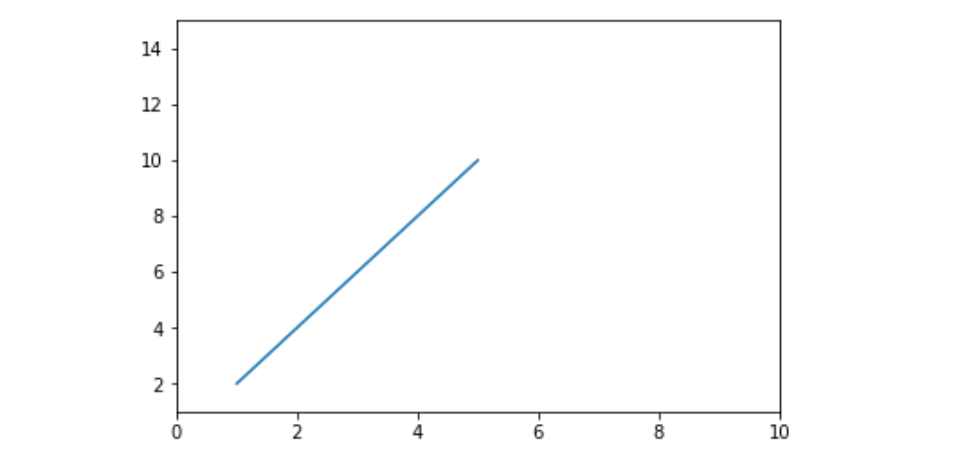Open in App
Not now

# Matplotlib.pyplot.axis() in Python

• Last Updated : 12 Apr, 2020

Matplotlib is a plotting library for creating static, animated, and interactive visualizations in Python. Pyplot is a Matplotlib module which provides a MATLAB-like interface. Matplotlib is designed to be as usable as MATLAB, with the ability to use Python and the advantage of being free and open-source.

## matplotlib.pyplot.axis()

This function is used to set some axis properties to the graph.

Syntax: matplotlib.pyplot.axis(*args, emit=True, **kwargs)

Parameters:
xmin, xmax, ymin, ymax:These parameters can be used to
set the axis limits on the graph
emit:Its a bool value used to notify observers of the axis limit change

Example #1:

 `import` `matplotlib.pyplot as plt`` ` ` ` `x ``=``[``1``, ``2``, ``3``, ``4``, ``5``]``y ``=``[``2``, ``4``, ``6``, ``8``, ``10``]`` ` `# Plotting the graph``plt.plot(x, y)`` ` `# Setting the x-axis to 1-10``# and y-axis to 1-15``plt.axis([``0``, ``10``, ``1``, ``15``])`` ` `# Showing the graph with updated axis``plt.show()`

Output:Example #2:

 `import` `matplotlib.pyplot as plt`` ` `x ``=``[``1``, ``2``, ``3``, ``4``, ``5``]``y ``=``[``2``, ``4``, ``6``, ``8``, ``10``]`` ` `plt.plot(x, y)`` ` `# we can turn off the axis and display``# only the line by passing the ``# optional parameter 'off' to it``plt.axis(``'off'``)`` ` `plt.show()`

Output:My Personal Notes arrow_drop_up